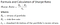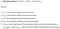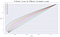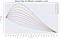# Visualizing the benefits of diversification with Python

Have you been tempted to put all you money on one hyped up stock, get rich and quit your job? If your answer is yes, this article is for you.

# Why is diversification a no-brainer?

We all (hopefully) know that smart diversification is an essential part of a good investment plan. However, when we see assets skyrocketing, it is tempting to want to put all you eggs in one basket.

But here is why you should always diversify:

## Risk vs Reward Trade-off

Before we continue, bear in mind that I will be using the terms volatility, risk and standard deviation interchangeably. Note that in portfolio analysis, they mean the same thing.

Let’s imagine that you have some cash to invest. You do some research and find two stocks that you find promising: GameStep and Nukia.

`### characteristics of each assetgame_step = {'expected_return': 0.25, 'volatility': 0.15}nukia = {'expected_return': 0.15, 'volatility': 0.07}correlation = 0.3`

## Sharpe Ratio

Well, if you had to choose just one, a good way to decide is to look at Sharpe Ratio of each one. It is a benchmark measure of the most important trade-off in investments: risk vs returnStandard Deviation = Risk = Volatility

To simplify our example, let’s just ignore the risk-free rate and calculate the Sharpe Ratio for each stock:

GameStep: 25% / 15% = 1.66

Nukia: 15% / 7% = 2.14

Out of these two, Nukia clearly offers better returns per risk, even though its expected return is lower than GameStep’s.

But what if you could allocate your cash on both assets? How much should you allocate in each one?

For that you would need to consider the combined returns and the combined risk of the portfolio.

## Portfolio Return

Let’s start off with the combined returns. It is very easy to calculate, since it will be the weighted average of the returns of GameStep and Nukia.

In other words, the portfolio return is a linear combination of both stocks returns.

In Python, you could calculate it as follows:

`def calculate_portfolio_returns(weight_a, weight_b):    ret_a = weight_a * game_step['expected_return']    ret_b = weight_b * nukia['expected_return']    combined_return = ret_a + ret_b    return combined_return`

## Portfolio Risk

On the other hand, the portfolio volatility is not a simple weighted average. If you diversify, it will be lower than the simple weighed average as long as both assets are not perfectly correlated.

The reason is that, unless both assets are perfectly correlated, each asset price will vary but not necessarily in the same way. When GameStep goes down by 5% , Nukia might be only 3% down, or even going up.

In other words, the variations of one asset will tend to offset the variations of the other at least to some degree, as long as they are not perfectly correlated. This is why the chart above does not show a straight line.

The result is that your risk (volatility) vs reward (returns) trade-off will be better: portfolio returns will be the average of individual returns, but the risk will be lower than the average of individual risks.

This becomes clearer when you look at the portfolio return and volatility against each other

Notice that they diverge right at the start. While returns grows linearly from 100% allocation on Nukia (lower return) to 100% allocation on GameStep (higher return), the same does not happen to the volatility.

This divergence is reflected on the Sharpe Ratio of the portfolio, shown in the bottom of the chart. The larger the distance between the straight line of the returns and the “curved” volatility, the higher the Sharpe Ratio.

Notice how the Sharpe Ration starts off at 2.14, which reflects 100% allocation on Nukia. It then grows and peaks at around 23% Nukia and 77% GameStep.

Even though Nukia offers a better Sharpe Ration as a stand alone investment, the ratio of the portfolio improves as we allocate a little on GameStep.

## Let’s get technical

If you want to go deeper into it, here is the formula for the portfolio variance (feel free to skip to the next session if you prefer):You don`t need to memorize it. The takeaway is that portfolio volatility is not a linear combination of the assets volatility.

Since the portfolio variance is equivalent to the portfolio volatility (standard deviation) squared, we just need to take the square root of the formula above to get our portfolio volatility.

Here the equivalent in Python code:

`# creates list of 21 diferent ratio combinations allocation_strategies = [[w, 1-w] for w in np.linspace(0, 1, 21)]# calculates the portfolio volatility correlation = 0.3def calculate_portfolio_vol(weight_a, weight_b, correlation):    vol_a = game_step['volatility']    vol_b = nukia['volatility']    portf_variance_formula =  (           ( weight_a**2 ) * (vol_a**2)        + ( weight_b**2 ) * (vol_b**2 )        + 2*correlation*weight_a*weight_b*vol_a*vol_b                              )                                  portf_volatility = portf_variance_formula**(1/2)    return portf_volatility`

And here is some more code that shows how to produce the charts above:

`# creates list of different allocation strategiesallocation_strategies = [[w, 1-w] for w in np.linspace(0, 1, 21)]# calculates the portfolio return and volatility # for each allocation strategyimport pandas as pddef test_allocation(allocation_strategies, correlation):    d = {}    portfolio_returns = []    portfolio_volatility = []    for allocation in allocation_strategies:        weight_a = allocation        weight_b = allocation                        combined_return = calculate_portfolio_returns(            weight_a,             weight_b)                portfolio_returns.append(combined_return)                combined_vol = calculate_portfolio_vol(            weight_a,             weight_b,             correlation)                portfolio_volatility.append(combined_vol)        d['returns'] = portfolio_returns    d['volatility'] = portfolio_volatility    return pd.DataFrame(d)portfolios_df = test_allocation(allocation_strategies, correlation)## plots the figures# creates figure and subplotsfig, axs = plt.subplots(10, 10,                         figsize=(10, 10),                         sharex=True)ax1 = plt.subplot2grid(shape=(10, 7),                        loc=(0,0),                        colspan=10,                        rowspan=7)ax3 = plt.subplot2grid(shape=(10, 3),                        loc=(7,0),                        colspan=10,                        rowspan=3)### plots the portfolio returns curvecolor = 'tab:blue'ax1.set_ylabel('Returns', color = color)  ax1.plot(alloc_labels,          portfolios_df['returns'],          color = color)  ax1.tick_params(axis ='y',                 labelcolor = color)ax1.tick_params(axis ='x',                 labelcolor = 'white')  # Adding Twin Axes to plot portfolio volatility on the same subplot as ax1 ax2 = ax1.twinx()### plots the portfolio returns curvecolor = 'tab:green'ax2.set_ylabel('Volatility',                color = color)  ax2.plot(alloc_labels,          portfolios_df['volatility'], color = color)  ax2.tick_params(axis ='y',                 labelcolor = color)# Adding title plt.title('Risk/Return for different allocation strategies',           fontweight ="bold")ax3.plot(alloc_labels,             portfolios_df['sharpe'],             color='gray',           label='Sharpe Ratio')ax3.set_ylabel('Sharpe Ratio', color = 'black') ax3.tick_params(axis ='y', labelcolor = 'black')ax3.tick_params(axis ='x', labelcolor = 'gray', rotation=45,)ax3.set_xlabel('allocation strategies')  plt.show()`

## Visualizing the Portfolio Curve

To get a better picture of how the portfolio performs for each allocation strategy, consider the chart below, that shows volatility (x-axis) and returns (y-axis):

Pay attention to the very beginning of the curve, at the red point (total allocation on Nukia, btw). What happens if you start to move up along the curve now? Volatility decreases while return increases, at least until a certain point. In fact, there is a blue point just above it that represents a possible allocation with the same volatility, but higher return.

All of this is due to how ‘warped’ this curve is.

Bottom line: you would neve want to be invested 100% on Nukia if you could have the same volatility and higher returns just by allocating a little on GameStep.

But wait, it gets better!

Look how the portfolio curve becomes more ‘warped’ as we change the correlation:the lower the correlation, the more “curved” it becomes

Notice that the lower the correlation, the more pronounced the ‘warp’ becomes, and the bigger the incentives to diversification.

The same thing happens to the Sharpe Ratio curve:The lower the correlation, the higher the maximum Sharpe becomes.

Obviously, you don’t choose the correlation between two assets, by you can choose assets that have low correlation. This is a key aspect to bear in mind.

Not convinced? Well, next week I will expand more on the issue, and show the same concepts, but for multiple assets.

To wrap up, here is the code for the last few charts:

`# portfolio curve chartplt.figure(figsize=(12, 7))plt.plot(portfolios_df['volatility'],         portfolios_df['returns'], color='purple'        )plt.title('Portfolio Curve', fontsize=20)plt.xlabel('Volatility')plt.ylabel('Return')plt.scatter(nukia['volatility'], nukia['expected_return'], color='red')plt.scatter(nukia['volatility'], 0.1665            , color='blue')plt.show()# portfolio curves for different correlationsplt.figure(figsize=(12, 7))for corr in np.linspace(0.1, 1, 10):    corr_df = test_allocation(allocation_strategies, corr)        plt.plot(corr_df['volatility'],         corr_df['returns'], label=round(corr, 1)        )plt.title('Portfolio Curves for Different Correlation Levels', fontsize=20)plt.xlabel('volatility')plt.ylabel('return')plt.legend()plt.show()# sharpe ratios for different correlationsplt.figure(figsize=(12, 7))for corr in np.linspace(0.1, 1, 10):    corr_df = test_allocation(allocation_strategies, corr)        plt.plot(alloc_labels,         corr_df['sharpe'], label=round(corr, 1)        )plt.title('Sharpe Ratio for Different Correlation Levels', fontsize=20)plt.tick_params(axis='x', rotation=45)plt.xlabel('allocation')plt.ylabel('Sharpe Ratio')plt.legend()plt.show()`

Data Scientist, Economist with a background in Banking www.linkedin.com/in/felipecezar1

## More from Felipe Cezar

Data Scientist, Economist with a background in Banking www.linkedin.com/in/felipecezar1